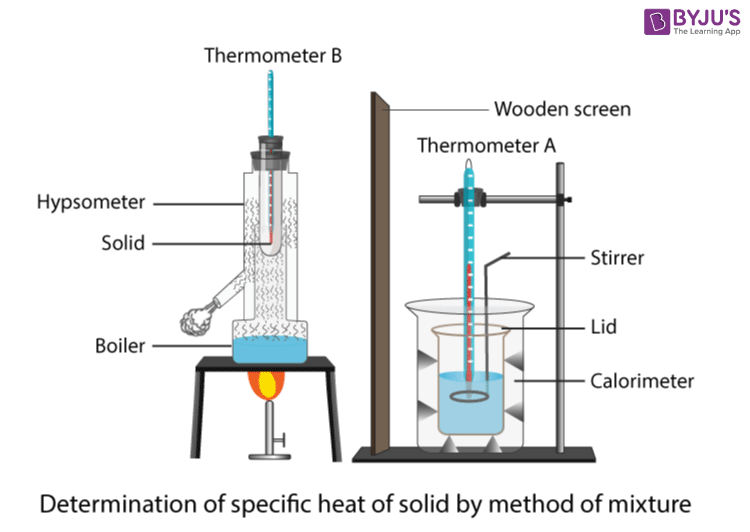# To Determine Specific Heat Capacity of a Given Solid by Method of Mixture

Specific heat capacity is defined as the amount of heat required for one gram of substance at 1℃. To understand how to determine the specific heat capacity of a given solid by the method of mixtures, please read the below article.

## Aim

To determine the specific heat capacity of a given solid by the method of mixtures.

## Materials Required

1. A hypsometer
2. Calorimeter
3. Stirrer
4. A lid and outer jacket
5. Solid in small pieces
6. Balance
7. Weight box
8. Two half-degree thermometers
9. Coldwater
10. Clamp stand

## Theory

The calorimeter is a device used to measure the heat flow of a chemical or physical reaction. Calorimetry is the process of measuring this heat. It consists of a metal container to hold water above the combustion chamber and a thermometer to measure the temperature change. Hypsometer is an instrument used to determine the boiling point of water at a given altitude.

## Diagram## Procedure

1. Put thermometers A and B in a beaker containing water and note their reading. Let thermometer A be a standard to find the correction that is to be applied to thermometer B.
2. Put the thermometer B into the copper tube of a hypsometer containing the powder of the given solid. Before placing the hypsometer on the burner, add a sufficient amount of water.
3. Record the weight of the calorimeter with a stirrer and lid over it.
4. Add water (temperature between 5 to 8℃) to the calorimeter at half-length and weigh it again.
5. Heat the hypsometer till the temperature of the solid is steady.
6. Note the temperature of water in calorimetry. Now slowly stir and add the solid powder from the hypsometer to the calorimeter and record the final temperature of the mixture.
7. Remove thermometer A from the calorimeter.
8. Note the weight of the calorimeter with the contents and lid.

## Observations

Reading of thermometer A = TA = ……… ℃

Reading of thermometer B = TB = ……… ℃

Correction applied in B with respect to A (TA – TB) = …….. ℃

Mass of calorimeter and stirrer m = …….. g

Water equivalent of calorimeter ω = m × 0.095 = …….. g

Specific heat of copper calorimeter = 0.095 cal/g

Mass of calorimeter + stirrer + lid = m1 = ……. g

Mass of calorimeter + stirrer + lid + cold water = m2 = …… g

The steady temperature of hot solid = Ts = …….. ℃

Corrected temperature of hot solid T =Ts – (TA – TB) = ……… ℃

The temperature of cold water = t =……… ℃

The temperature of the mixture = Ө =…….. ℃

Mass of calorimeter, stirrer, lid, cold water, and solid = m3 =…… g

## Calculations

Mass of cold water = m2 – m1 =…….. g

Mass of hot solid = m3 – m2 =…….. g

Rise of the temperature of cold water and calorimeter = Ө – t =………. ℃

Fall in temperature of solid = T – Ө =……… ℃

Heat gain by calorimeter, cold water and stirrer = [ω + ( m2 – m1)(Ө – t)] =……. (a)

Heat lost by solid = (m3 – m2) × C × (T – Ө) =……. (a)

Here, C is the specific heat of solid to be calculated.

According to the principle of calorimeter, heat lost = heat gained

(m3 – m2) × C × (T – Ө) = [ω + ( m2 – m1)(Ө – t)]

$$\begin{array}{l}C=\frac{[\omega +(m_{2}-m_{1})(\theta -t)]}{[(m_{3}-m_{2})(T-\theta )]}=…..\;cal/g^{0}C\end{array}$$

## Result

Specific heat of given solid by the method of the mixture is ………. cal g-1-1.

## Precautions

1. A sufficient amount of solid powder should be used to cover the tip of the thermometer.
2. A sufficient amount of water should be taken in a hypsometer.
3. Dropping of solid should be quick and gentle.
4. To avoid excess radiation, the calorimeter should be polished from the outside.
5. The temperature of cold water should not cross the dew point.

## Sources of Error

1. There might be heat loss while transferring solid into the calorimeter.
2. During conduction, convection, and radiation, there might be heat loss.
3. The bulbs of the thermometer might not be completely inside the solid.

Note: To determine the specific heat of a given liquid by the method of mixtures, the liquid is taken in place of cold water to determine the specific heat. Proceed with the same procedure as in the experiment.

## Viva Voice

Q1. What is the heat?

Ans: Heat is defined as the quality of being hot at high temperatures.

Q2. Define the specific heat of the substance.

Ans: It is defined as the amount of heat energy required to raise the temperature of 1 gram of a substance by 1℃.

Q3. State the principle of calorimetry.

Ans: The principle of calorimetry is heat lost is equal to the heat gained.

Q4. Why is calorimeter made of copper is used in the experiment?

Ans: The specific heat of copper is very low. The absorption and liberation of heat will be less during heat transfer.

Q5. Is heat gained always equal to the heat lost?

Ans: If there is no chemical reaction taking place between the components only then heat loss will be always equal to the heat gain.